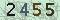﻿Robust and efficient estimation for thetreatment effect in causal inference and missing data problems-吉林大学新闻网## Robust and efficient estimation for thetreatment effect in causal inference and missing data problems

The Mann-Whitney statistic based on complete data only might beinvalid if the outcome variables are missing due to certain covariates. In thispaper, we used the probabilistic index modelling to obtain a new Mann-Whitneystatistic when missingness occurs in the outcomes but multiple explanatoryvariables are observable. Our method combined the efficiency of the model-basedapproach and the robustness of the nonparametric approach. It requires fewmodel assumptions and is shown to be efficient if all specifications arecorrect, and doubly robust if some part is misspecified. Results fromsimulation studies and a real data analysis of consumer phone loans in Chinaare presented to demonstrate the advantages of the proposed method over othermethods.

Inthis talk, we mainly study derivations, biderivations and triple derivations onJordan algebras.Firstly, the sufficient and necessary conditions for theirderivation algebras being simple are given. As an application, triplederivations of derivation algebras of semi-simple Jordan algebras are studied.Then we give a theorem about the relationship between biderivations andcentroid of Jordan algebras and show that triple derivations are allderivations on Jordan algebras under some assumptions. Moreover, we also give atheorem about triple homomorphisms on Jordan algebras.

We give a characterization of random Toeplitz operators as randomlinear operators on Hilbert spaces given by multiplications. Their propertiesare studied in term of their generating functions. Our results, whenapplied with the preconditioned conjugate gradient method, gives anew technique for finding numerical solutions of linearsystems associated with a random Toeplitz coefficient matrices. We alsoinvestigate the Weyl equidistribution of eigenfunctions of random Toeplitzmatrices.This is a joint work with King-Fai Lai, Tsung-Lin Lee and Wen-Fong Ke.

In this talk, Iwill introduce some recent results about linear preservers on some functionspaces. In particular, I will talk about the order isomorphism ondifferentiable function spaces, the inverse of disjointeness preserving maps,and weak local isometries on uniform algebras.

In this talk, we introducesome recent results concerning reducing subspaces for Toeplitz operators onBergman type spaces. We characterize the minimal reducing subspaces forToeplitz operator with quasi-homogeneous symbol, and give several interestingexamples on the Bergman type spaces over bidisk and disk respectively. Finally,we state these results in terms of the commutant algebra.

 我要评论： 匿名发布 验证码看不清楚,换张图片 共0条评论    共1页   当前第1页

### 相关文章

• 读取内容中,请等待...

E-mail:jlunewsnet@163.com
••